SLVSDA7E February   2017  – August 2019

PRODUCTION DATA.

1. Features
2. Applications
3. Description
1.     Device Images
4. Revision History
5. Device Comparison Table
6. Pin Configuration and Functions
7. Specifications
8. Detailed Description
1. 8.1 Overview
2. 8.2 Functional Block Diagram
3. 8.3 Feature Description
4. 8.4 Device Functional Modes
9. Application and Implementation
1. 9.1 Application Information
2. 9.2 Typical Application
1. 9.2.1 Design Requirements
2. 9.2.2 Detailed Design Procedure
3. 9.2.3 Setting the Current Limit
4. 9.2.4 Setting the Output Voltage
5. 9.2.5 TPS61178 Application Waveform
3. 9.3 System Examples
10. 10Power Supply Recommendations
11. 11Layout
12. 12Device and Documentation Support
1. 12.1 Device Support
2. 12.2 Documentation Support
5. 12.5 Community Resources
7. 12.7 Electrostatic Discharge Caution
8. 12.8 Glossary
13. 13Mechanical, Packaging, and Orderable Information

• RNW|13

#### 9.2.4.4.2 Loop Compensation Design Steps

With the small signal models coming out, the next step is to calculate the compensation network parameters with the given inductor and output capacitance.

1. Set the Cross Over Frequency, ƒC
• The first step is to set the loop crossover frequency, ƒC. The higher crossover frequency, the faster the loop response is. It is generally accepted that the loop gain cross over no higher than the lower of either 1/10 of the switching frequency, ƒSW, or 1/5 of the RHPZ frequency, ƒRHPZ. Then calculate the loop compensation network values of RC, CC, and CP by following below equations.
2. Set the Compensation Resistor, RC
• By placing ƒZ below ƒC, for frequencies above ƒC, RC | | REA ~= RC and so RC × GEA sets the compensation gain. Setting the compensation gain, KCOMP-dB, at ƒZ, results in the total loop gain, T(s) = GPS(s) × HEA(s) × He(s) being zero at ƒC.
• Therefore, to approximate a single-pole roll-off up to fP2, rearrange Equation 22 to solve for RC so that the compensation gain, KEA, at fC is the negative of the gain, KPS, read at frequency fC for the power stage bode plot or more simply:
• Equation 26.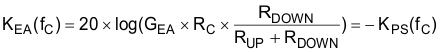where

• KEA is gain of the error amplifier network
• KPS is the gain of the power stage
• GEA is the amplifier’s trans-conductance, the typical value of GEA = 195 µA / V
3. Set the compensation zero capacitor, CC
• Place the compensation zero at the power stage ROUT ,COUT pole’s position, so to get:
• Equation 27.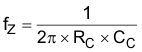• Set ƒZ = ƒP, and get the
• Equation 28.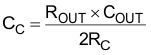4. Set the compensation pole capacitor, CP
• Place the compensation pole at the zero produced by the RESR and the COUT, it is useful for canceling unhelpful effects of the ESR zero.
• Equation 29.Equation 30.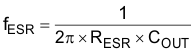• Set ƒP2 = ƒESR, and get the
• Equation 31.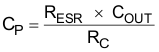• If the calculated value of CP is less than 10 pF, it can be neglected.
Designing the loop for greater than 45° of phase margin and greater than 6-dB gain margin eliminates output voltage ringing during the line and load transient. The RC = 15 kΩ , CC = 6.8 nF, Cp = 10 pF for this design example.